Triangular tiling

﻿
Triangular tiling
Triangular tiling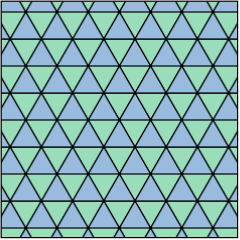Type Regular tiling
Vertex configuration 3.3.3.3.3.3 (or 36)
Schläfli symbol(s) {3,6}
{3}
Wythoff symbol(s) 6 | 3 2
3 | 3 3
| 3 3 3
Coxeter-Dynkin(s)Symmetry p6m, [6,3], *632
p3m1, [3], *333
p3, [3]+, 333
Dual Hexagonal tiling
Properties Vertex-transitive, edge-transitive, face-transitive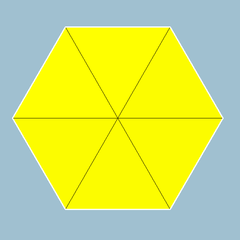3.3.3.3.3.3 (or 36)

In geometry, the triangular tiling is one of the three regular tilings of the Euclidean plane. Because the internal angle of the equilateral triangle is 60 degrees, six triangles at a point occupy a full 360 degrees. The triangular tiling has Schläfli symbol of {3,6}.

Conway calls it a deltille, named from the triangular shape of the greek letter delta (Δ). The triangular tiling is roughly the kishextile.

It is one of three regular tilings of the plane. The other two are the square tiling and the hexagonal tiling.

This vertex arrangement is called the A2 lattice.

Uniform colorings

There are 9 distinct uniform colorings of a triangular tiling. (Naming the colors by indices on the 6 triangles around a vertex: 111111, 111112, 111212, 111213, 111222, 112122, 121212, 121213, 121314)

Four of the colorings are generated by Wythoff constructions. Seven of the nine distinct colorings can be made as reductions of the four coloring: 121314. The remaining two, 111222 and 112122, have no Wythoff constructions.

Coloring Coloring Symmetry Wythoff symbol indices 111111 121212 121314 121213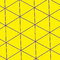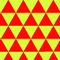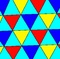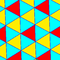*632 (p6m) [6,3] *333 (p3m1) [3] 333 (p3) [3]+ 3*3 (p31m) [6,3+] 6 | 3 2 3 | 3 3 | 3 3 3Coloring Coloring Symmetry indices 111222 112122 111112 111212 111213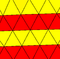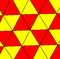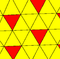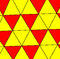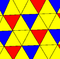2*22 (cmm) [∞,2+,∞] 2222 (p2) [∞,2,∞]+ *333 (p3m1) [3] *333 (p3m1) [3] 333 (p3) [3]+

Related polyhedra and tilings

The planar tilings are related to polyhedra. Putting fewer triangles on a vertex leaves a gap and allows it to be folded into a pyramid. These can be expanded to Platonic solids: five, four and three triangles on a vertex define an icosahedron, octahedron, and tetrahedron respectively.

This tiling is topologically related as a part of sequence of regular polyhedra with Schläfli symbols {3,n}, continuing into the hyperbolic plane.

It is also topologically related as a part of sequence of Catalan solids with face configuration Vn.6.6, and also continuing into the hyperbolic plane.

Wikimedia Foundation. 2010.

Look at other dictionaries:

• Order-7 triangular tiling — Poincaré disk model of the hyperbolic plane Type Regular hyperbolic tiling Vertex figure 37 Schläfli symbol(s) …   Wikipedia

• Order-7 truncated triangular tiling — Poincaré disk model Type Hyperbolic semiregular tiling Vertex figure 7.6.6 Schläfli symbol t{3,7} …   Wikipedia

• Elongated triangular tiling — In geometry, the elongated triangular tiling is a semiregular tiling of the Euclidean plane. There are three triangles and two squares on each vertex.Conway calls it a isosnub quadrille.There are 3 regular and 8 semiregular tilings in the… …   Wikipedia

• Triakis triangular tiling — Infobox face uniform polyhedron Polyhedron Type = Dual semiregular tiling Face List = triangle Edge Count = Infinite Vertex Count = Infinite Symmetry Group = p6m or *632 Face Type = V3.12.12 Dual = Truncated hexagonal tiling Property List = face… …   Wikipedia

• Triangular prismatic honeycomb — The triangular prismatic honeycomb is a space filling tessellation (or honeycomb) in Euclidean 3 space. It is comprised entirely of triangular prisms.It is constructed from a triangular tiling extruded into prisms.It is one of 28 convex uniform… …   Wikipedia

• Triangular-hexagonal prismatic honeycomb — The triangular hexagonal prismatic honeycomb is a space filling tessellation (or honeycomb) in Euclidean 3 space. It is comprised of hexagonal prisms and triangular prisms in a ratio of 1:2.It is constructed from a trihexagonal tiling extruded… …   Wikipedia

• Order-3 heptagonal tiling — Poincaré disk model of the hyperbolic plane Type Regular hyperbolic tiling Vertex figure 7.7.7 Schläfli symbol(s) …   Wikipedia

• Hexagonal tiling — In geometry, the hexagonal tiling is a regular tiling of the Euclidean plane. It has Schläfli symbol of {6,3} or t{3,6} (as a truncated triangular tiling).Conway calls it a hextille.The internal angle of the hexagon is 120 degrees so three… …   Wikipedia

• Order-3 truncated heptagonal tiling — Poincaré disk model Type Hyperbolic semiregular tiling Vertex figure 3.14.14 Schläfli symbol t{7 …   Wikipedia

• Snub square tiling — In geometry, the snub square tiling is a semiregular tiling of the Euclidean plane. There are three triangles and two squares on each vertex. It has Schläfli symbol of s{4,4} .Conway calls it a snub quadrille, constructed as a snub operation… …   Wikipedia